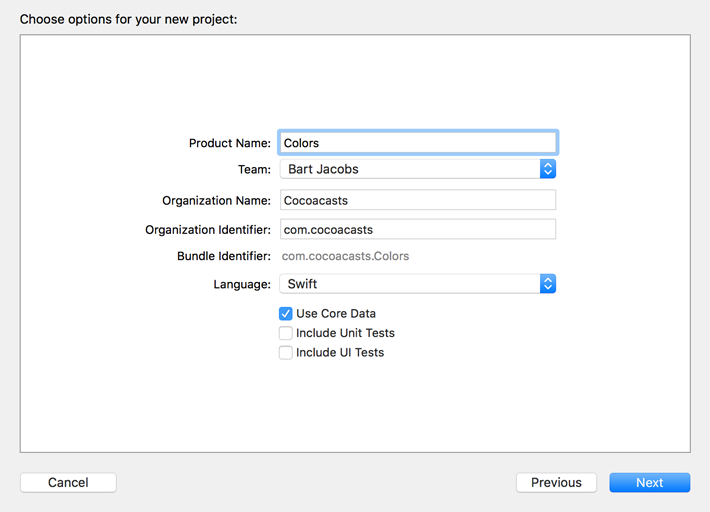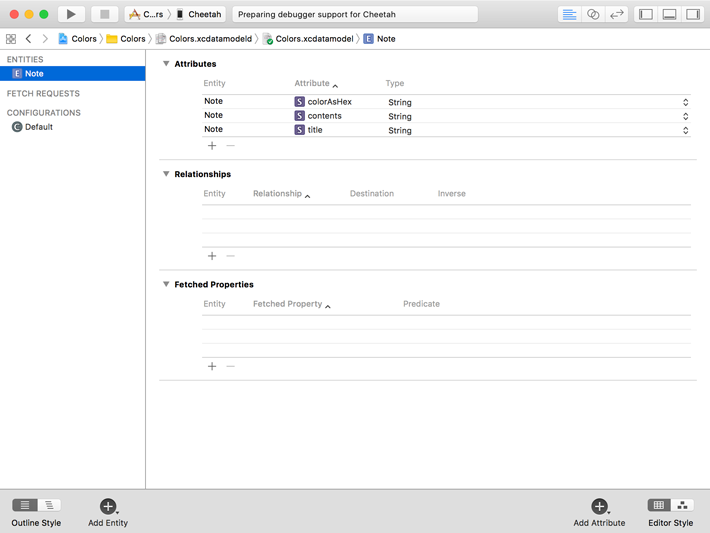# How to Store UIColor in Core Data Persistent Store

The types you can store in a Core Data persistent store are limited and it probably won't surprise you that `UIColor` objects are not supported out of the box.

You could use transformable attributes, but I prefer an alternative approach for storing colors. In this tutorial, I show you how to store `UIColor` objects in a Core Data persistent store. This is a topic we also we cover in Core Data Fundamentals.

## Project Setup

Fire up Xcode and create a new project based on the iOS > Single View App template.Name the project Colors and set Language to Swift. To save us some time, we also check Use Core Data at the bottom.Save the project and select the data model of the project, Colors.xcdatamodeld. Create a new entity and name it Note. Add three attributes to the Note entity.

• title of type String
• contents of type String
• colorAsHex of type StringEach note can have a title, contents, and a color. As the name of the colorAsHex attribute suggests, we store the color as a string, a hex value. One of the advantages is that it's easy to debug. It's easier to read hex values than binary data. Right?

We don't stop here, though. We don't want to work with hex values. The goal is to only interact with `UIColor` instances. Converting a `UIColor` instance to a hex value and vice versa should be handled for us, automatically.

## Extensions to the Rescue

As of Xcode 8, the class definition of the Note entity is automatically created for us. But we want to add some functionality to the `Note` class to make it easier to work with colors.

### Extending UIColor

We first create an extension for the `UIcolor` class. In the extension, we define a convenience initializer for creating a `UIColor` instance from a hex value. I explain the implementation in more detail in another tutorial.

``````import UIKit
import Foundation

extension UIColor {

// MARK: - Initialization

convenience init?(hex: String) {
var hexNormalized = hex.trimmingCharacters(in: .whitespacesAndNewlines)
hexNormalized = hexNormalized.replacingOccurrences(of: "#", with: "")

// Helpers
var rgb: UInt32 = 0
var r: CGFloat = 0.0
var g: CGFloat = 0.0
var b: CGFloat = 0.0
var a: CGFloat = 1.0
let length = hexNormalized.characters.count

// Create Scanner
Scanner(string: hexNormalized).scanHexInt32(&rgb)

if length == 6 {
r = CGFloat((rgb & 0xFF0000) >> 16) / 255.0
g = CGFloat((rgb & 0x00FF00) >> 8) / 255.0
b = CGFloat(rgb & 0x0000FF) / 255.0

} else if length == 8 {
r = CGFloat((rgb & 0xFF000000) >> 24) / 255.0
g = CGFloat((rgb & 0x00FF0000) >> 16) / 255.0
b = CGFloat((rgb & 0x0000FF00) >> 8) / 255.0
a = CGFloat(rgb & 0x000000FF) / 255.0

} else {
return nil
}

self.init(red: r, green: g, blue: b, alpha: a)
}
}
``````

We also implement a computed property for converting a `UIColor` instance to its corresponding hex value.

``````import UIKit
import Foundation

extension UIColor {

// MARK: - Initialization

convenience init?(hex: String) {
...
}

// MARK: - Convenience Methods

var toHex: String? {
// Extract Components
guard let components = cgColor.components, components.count >= 3 else {
return nil
}

// Helpers
let r = Float(components)
let g = Float(components)
let b = Float(components)
var a = Float(1.0)

if components.count >= 4 {
a = Float(components)
}

// Create Hex String
let hex = String(format: "%02lX%02lX%02lX%02lX", lroundf(r * 255), lroundf(g * 255), lroundf(b * 255), lroundf(a * 255))

return hex
}

}
``````

### Extending Note

The last piece of the puzzle is surprisingly easy. We create an extension for the `Note` class and define a computed property, `color`, of type `UIColor?`.

``````import UIKit
import Foundation

extension Note {

var color: UIColor? {}

}
``````

We implement the getter and setter of the computed property to convert the value of the `colorAsHex` property. In the getter, we convert the value of the `colorAsHex` property to a `UIColor` instance. We use the convenience initializer we implemented earlier.

``````import UIKit
import Foundation

extension Note {

var color: UIColor? {
get {
guard let hex = colorAsHex else { return nil }
return UIColor(hex: hex)
}
}

}
``````

In the setter, we convert the new value of the computed property to a string using the `toHex` computed property we implemented a moment ago. We store the converted value in the `colorAsHex` property.

``````import UIKit
import Foundation

extension Note {

var color: UIColor? {
get {
...
}
set(newColor) {
if let newColor = newColor {
colorAsHex = newColor.toHex
}
}
}

}
``````

The result is that we don't directly access the `colorAsHex` property of the `Note` class. Instead, we use the `color` computed property as a proxy. The `color` computed property is in charge of value conversion.

``````// Getting a Color
let color = note.color

// Setting a Color
note.color = UIColor(hex: "FF5F5B")
``````